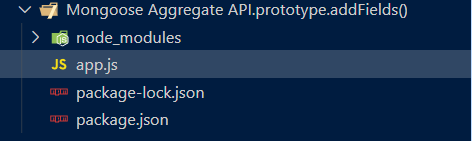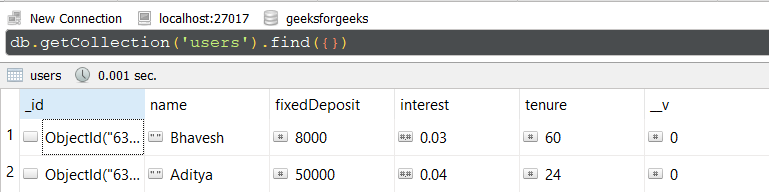Open in App
Not now

• Last Updated : 08 Dec, 2022

The Aggregate API.prototype.addFields() method of the Mongoose API is used to perform aggregation task. It allows us to add new fields to the output documents. Using addFields() method we can add custom fields to the result set along with the existing fields and can see them in the output documents.

Syntax:

`aggregate(.....).addFields( Object )`

Parameters: This method accepts a single parameter as described below:

• Object: It is the object that will be used to specify new fields we want to include in the result set.

Return Value: This method returns the result set in the form of an array.

Setting up Node.js Mongoose Module:

Step 1: Create a Node.js application using the following command:

`npm init`

Step 2: After creating the NodeJS application, Install the required module using the following command:

`npm install mongoose`

Project Structure: The project structure will look like this:Database Structure: The database structure will look like this, the following documents are present in the collection.Example 1: In this example, we have established a database connection using mongoose and defined model over userSchema, having five columns or fields “_id”, “name”, “fixedDeposit”, “interest”, and “tenure”. At the end, we are calling addFields() method and adding one new field to output document along with existing fields. Name of  the new field is returnOnInvestment this field is calculating the return amount on investment by multiplying principal amount, interest rate and tenure.

Filename: app.js

## Javascript

 `// Require mongoose module ` `const mongoose = require(``"mongoose"``); ` ` `  `// Set Up the Database connection ` `mongoose.connect(``"mongodb://localhost:27017/geeksforgeeks"``, { ` `    ``useNewUrlParser: ``true``, ` `    ``useUnifiedTopology: ``true``, ` `}); ` ` `  `const userSchema = ``new` `mongoose.Schema({ ` `    ``name: String, ` `    ``fixedDeposit: Number, ` `    ``interest: Number, ` `    ``tenure: Number ` `}); ` ` `  `const User = mongoose.model(``'User'``, userSchema); ` ` `  `User.aggregate() ` `    ``.project({ name: 1, fixedDeposit: 1, interest: 1, tenure: 1 }) ` `    ``.addFields({ ` `        ``returnOnInvestment: { ` `            ``\$multiply: [``"\$fixedDeposit"``, ``"\$interest"``, ``"\$tenure"``] ` `        ``} ` `    ``}).then(result => { ` `        ``console.log(result) ` `    ``})`

Step to run the program: To run the application execute the below command from the root directory of the project:

`node app.js`

Output:

```[
{
_id: new ObjectId("638f0262cc8a382bcf3d93df"),
name: 'Bhavesh',
fixedDeposit: 8000,
interest: 0.03,
tenure: 60,
investmentReturn: 14400
},
{
_id: new ObjectId("638f0262cc8a382bcf3d93de"),
fixedDeposit: 50000,
interest: 0.04,
tenure: 24,
investmentReturn: 48000
}
]```

Example 2: In this example, we have established a database connection using mongoose and defined model over userSchema, having five columns or fields “_id”, “name”, “fixedDeposit”, “interest”, and “tenure”. At the end, we are calling addFields() method and adding one new field to output document along with existing fields. Name of new field is oneMonthReturn this field is calculating the return amount on investment for one month by multiplying principal amount, interest rate by 1.

Filename: app.js

## Javascript

 `// Require mongoose module ` `const mongoose = require(``"mongoose"``); ` ` `  `// Set Up the Database connection ` `mongoose.connect(``"mongodb://localhost:27017/geeksforgeeks"``, { ` `    ``useNewUrlParser: ``true``, ` `    ``useUnifiedTopology: ``true``, ` `}); ` ` `  `const userSchema = ``new` `mongoose.Schema({ ` `    ``name: String, ` `    ``fixedDeposit: Number, ` `    ``interest: Number, ` `    ``tenure: Number ` `}); ` ` `  `const User = mongoose.model(``'User'``, userSchema); ` ` `  `User.aggregate([{ ` `    ``\$project: ` `        ``{ name: 1, fixedDeposit: 1, interest: 1, tenure: 1 } ` `}]) ` `    ``.addFields({ ` `        ``oneMonthReturn: ` `            ``{ \$multiply: [``"\$fixedDeposit"``, ``"\$interest"``, 1] } ` `    ``}).exec((error, success) => { ` `        ``if` `(error) ` `            ``console.log(error); ` `        ``else` `            ``console.log(success); ` `    ``})`

Step to run the program: To run the application execute the below command from the root directory of the project:

`node app.js`

Output:

```[
{
_id: new ObjectId("638f0262cc8a382bcf3d93df"),
name: 'Bhavesh',
fixedDeposit: 8000,
interest: 0.03,
tenure: 60,
oneMonthReturn: 240
},
{
_id: new ObjectId("638f0262cc8a382bcf3d93de"),